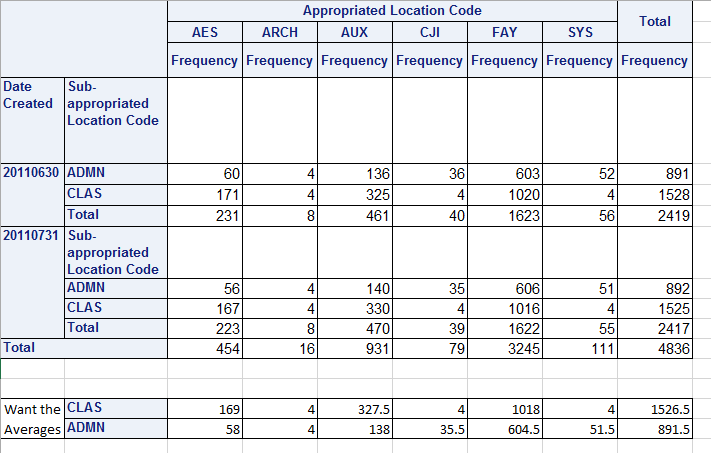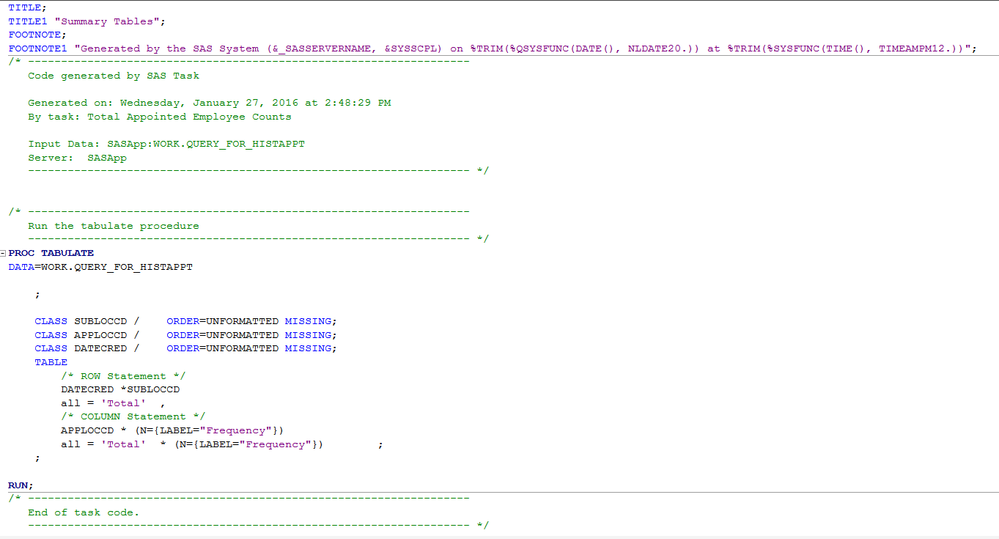## Calculating Average Number of Employees per month

Hello everyone,

I'm a new user of SAS and I'm learning how to use it more and more each day so please I apologize if this is a basic question.

I have built a process using SAS EG to help me identify turnover for our organization. In this process I need to calculate the total number of employees on average for a month. Below is a recap on what I have in the table:

PROC SQL;
CREATE TABLE WORK.QUERY_FOR_HISTAPPT AS
SELECT t1.DATECRED,
t1.DATECRED_DC,
t1.DATE_EFF,
t1.DATE_EFF_DC,
t1.LEGISTCD,
t1.OCC_CD,
t1.OCCTITLE,
t1.EMP_ID,
t1.EMPNAME,
t1.BUNITNO,
t1.BUNITCD,
t1.APPLOCCD,
t1.SUBLOCCD,
t1.POS_TYPE,
t1.STUDTITL,
t1.position
FROM HRMS.HISTAPPT t1
WHERE t1.DATECRED BETWEEN '20110630' AND '20110731' AND t1.EMP_ID NOT = '000000' AND t1.STUDTITL NOT = 'Y' AND
t1.OCC_CD NOT IN
(
'2903',
'2472',
'2902',
'2460',
'2441',
'2442',
'2476',
'2443',
'2478',
'2950',
'2901',
'2487',
'2907',
'2471',
'2479',
'2464',
'2465',
'2473',
'2440',
'2463',
'2445',
'2448',
'2908',
'2906',
'2475',
'2904',
'2474',
'2461',
'2462'
) AND t1.SUBLOCCD NOT = 'ACAD';
QUIT;

Ok, So I am pulling in all employees in an appointed positions at the end of two months, so all records are in this one table. Now I want to see if I can get the average number of employees by position type for that timeframe. I can get the actual numbers in a summary table; however, the average is really what I need. Any help would be greatly appreciated!

Thanks,

Shannon

8 REPLIES 8

## Re: Calculating Average Number of Employees per month

What are you defining as average? Over the two months?

Please post some sample data and expected output.

## Re: Calculating Average Number of Employees per month

Here's what I'm doing currently, I'm taking the summary table and dumping it into excel and then calculating the average by taking the total number of employees at the end of the two months, adding them together then dividing the total by 2.

=(total employees on 20110731) + (total employees on 20110831))/2

I have attached an image of the summary table.

Shannon## Re: Calculating Average Number of Employees per month

But how are you generating that table? Is it through proc tabulate?

We need to see a sample of the data structure that you'd be working with in SAS.

## Re: Calculating Average Number of Employees per month

Okay, so when I look at the code for the summary table is does say Proc Tabulate. I am attaching a screenshot.## Re: Calculating Average Number of Employees per month

Ok

Try changing the statistics requested - I'm assuming you're using a table builder of sorts.

Where it has N, you can add Mean

(N={Label="Frequency"} Mean{Label="Mean"})

You can play around with it to see if generates what you need.

Thanks Reeza.

## Re: Calculating Average Number of Employees per month

After thinking about it, I don't think it will generate your answer. In fact, I'm not sure it's possible within proc tabulate 😞

## Re: Calculating Average Number of Employees per month

@slolive wrote:

Okay, so when I look at the code for the summary table is does say Proc Tabulate. I am attaching a screenshot.

Run the Tabulate code with an Out=SummaryData on the proc statement. This will create a data set with the monthly totals.

Use that data to calculate the mean. Note that the summary variables in the dataset will have suffixes such as _sum added to them, so you can't just use the exact same tabulate code. But it shouldn't be horrific.

If we had an actual input data set example we could provide more exact code example.

Discussion stats
• 8 replies
• 1894 views
• 0 likes
• 3 in conversation